# Solving Equations with Linear Interpolation

Click here to go to our main page on linear interpolation using Excel

In engineering there are tons of equations you will encounter that are "one-way", that is there is an important quantity that is stuck as independent variables. Examples in the microwave industry include microstrip, CPW and stripline equations for Z0; these equations use physical parameters such as line width and substrate height to calculate line impedance, but never the other way around. By the way, "heighth" is not a word, we want to pass that on to all of you hicks out there who are eating squirrel for dinner...

So what if you want to calculate the width for a line that is exactly fifty ohms, for a given substrate height and dielectric? This is something that's you'd need to do if you were designing a microstrip Klopfenstein taper for example. There's no way to solve this equation for W: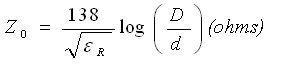where Eff is given by: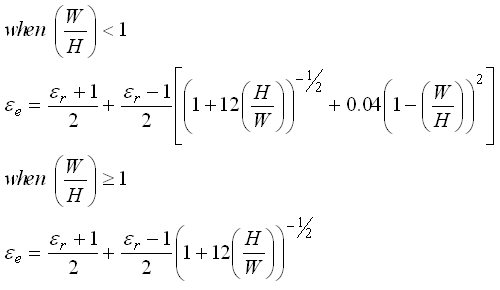Even Stephen Hawking couldn't come up with a closed form equation for W in terms of ER and H.

### Microsoft Excel's Solver

If you are a pencil-neck power-user of Excel, you'd know that there is a "solver toolpak" you can activate which can help. Basically, you select the cell you want to solve for, enter a value, and state which cell to vary to get there. Excel ponders the problem and comes up with the answer in short order. Nice work, if you can remember how to do it and enjoy clicking a your mouse a dozen times for each width value. Before you can try it you need to go to "tools", "add-ins" and check the solver add-in button.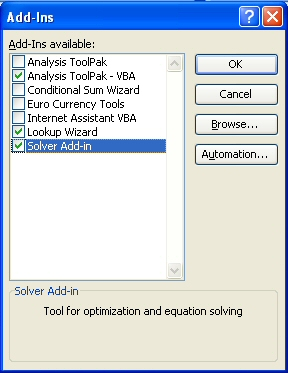Here's the solver in action. In this case you are solving cell B10 so that cell B5 results in a value of 0.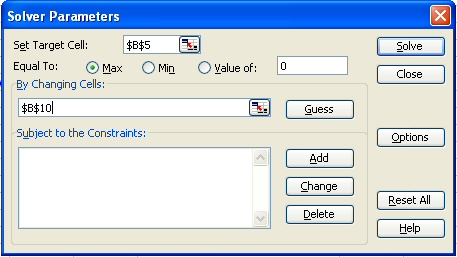Another choice would be to buy some expensive software, such as Agilent's Advanced Design System. It has a solver built into "Linecalc", which allows the user to apply transmission line impedance equations in both directions. Great if you have an extra \$50K laying about. We used to have that kind of loose cash, then we started this web site...

### Example: a reverse microstrip calculator

A more convenient way is to use Excel to generate a table of values for the one-way equation, the use out linear interpolation to pick off the value you are looking for.

We used the equations for microstrip Keff and Z0 from this page to create a table of impedance/width points. In the example shown below, 100um GaAs is the substrate, what you might encounter in a MMIC design. The spreadsheet table states the width value at H/100 (in this case 1um) all the way to HX10 (here it's 1mm). The increments are 500 geometrically incremented steps, probably overkill because the microstrip equations we're using aren't the most accurate! Below is a plot of the width/impedance data.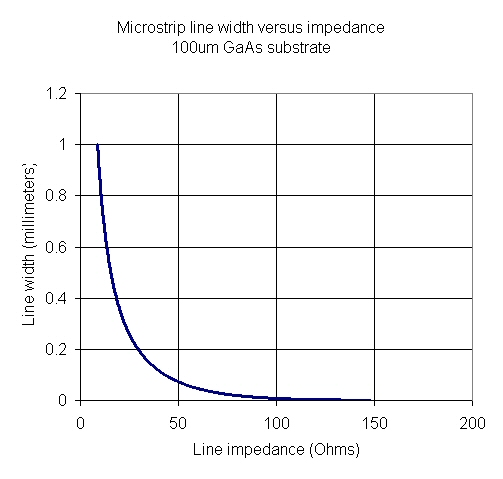The next step is to linearly interpolate width from impedance. For example, if you want 70.7 ohms, the nearest points in the table are 70.54 and 70.86 ohms which correspond to width values of 0. 029107171 and 0.028707806 millimeters. The spreadsheet does the heavy lifting and arrives at a value of 0.02890784 millimeters for 70.7 ohms. In practice we'd round that out to 28.9 or even 30 microns before going to layout. What is your degree in engineering worth if it doesn't provide the ability to ignore small errors?